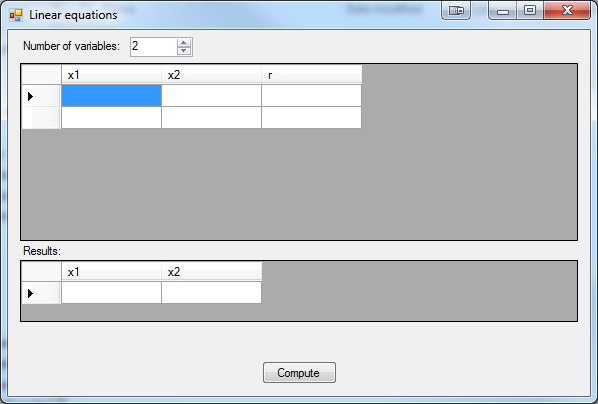### Features And Algorithms

Linear equations library uses Gaussian elimination method to compute variables.

### Examples And Usage

Computing simultaneous equations:

x1 + 3*x2 - x3 + 7*x4 = 17
2*x1 + 8*x2 + 3*x3 - 3*x4 = -9
3*x1 + 6*x2 - 2*x3 + 5*x4 = 8
4*x1 + 3*x2 + 7*x3 - 2*x4 = 9

will look like this:
`double[,] coefficients = new double[4,5];coefficients[0, 0] = 1;coefficients[0, 1] = 3;coefficients[0, 2] = -1;coefficients[0, 3] = 7;coefficients[0, 4] = 17;coefficients[1, 0] = 2;coefficients[1, 1] = 8;coefficients[1, 2] = 3;coefficients[1, 3] = -3;coefficients[1, 4] = -9;coefficients[2, 0] = 3;coefficients[2, 1] = 6;coefficients[2, 2] = -2;coefficients[2, 3] = 5;coefficients[2, 4] = 8;coefficients[3, 0] = 4;coefficients[3, 1] = 3;coefficients[3, 2] = 7;coefficients[3, 3] = -2;coefficients[3, 4] = 9;LinearEquation gauss = new LinearEquation(coefficients);double[] result = gauss.Compute();string output = string.Empty;for (int i = 0; i < 4; i++)    output += "x" + (i + 1) + " = " + result[i] + Environment.NewLine;`

Output:
`x1 = 1x2 = -1x3 = 2x4 = 3`

### Example application